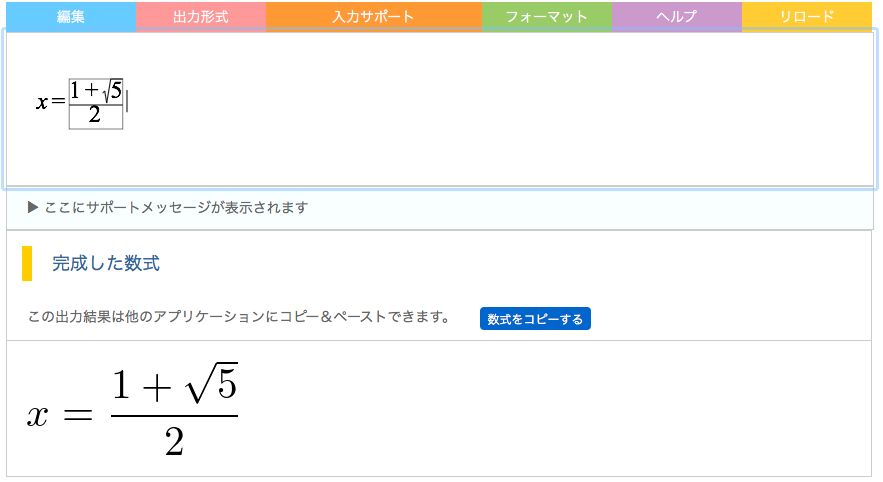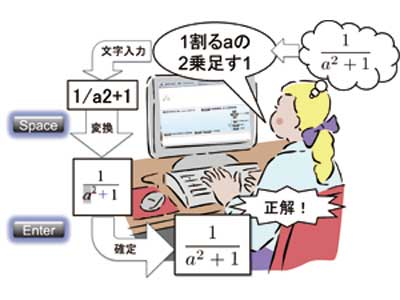### MathTOUCHIn 2011, we developed a math input editor, named MathTOUCH, that facilitates conversion from a colloquial-style mathematical text. In this method, the list of candidates for the desired mathematical expression is shown in WYSIWYG based on the userʼs input. After all the elements that a user wishes to use are chosen interactively, the desired expression can be formed. This method enables the user to input almost any mathematical formula without learning a new language or syntax.

### How to use it?• Step 1: Touch the colloquial-style mathematical text(string).
• Step 2: Choose the desired element from the list of candidates.
• Step 3: Paste into another application in various formats.(LaTeX, MathML, JPEG, PNG, EPS, Maxima, Mathmatica, Maple)

The rules for a linear mathematical string for a mathematical expression are described as follows:

Set the key letters (or words) corresponding to the elements of a mathematical expression linearly in the order of the colloquial (or reading) style, without considering two-dimensional placement and delimiters.

In other words, a key letter (or word) consists of the ASCII code(s) corresponding to the initial or the clipped form (such as the LaTeX-form) of the objective mathematical symbol. Therefore, a single key often supports many mathematical symbols.For example, when a user wants to input $$\alpha^2$$, the linear string is denoted by “a2”, where “a” represents the “alpha” symbol and it is unnecessary to include the power sign (i.e., the caret letter (\^{})).
In the case of $\frac{1}{\alpha^2+3}$, the linear string is denoted by “1/a2+3”, where it is not necessary to put the denominator (which is generally the operand of an operator) in parentheses, because those are never printed.
After all the elements that a user wishes to use are chosen interactively, the desired expression can be formed.
The output expression is able to paste into a document with another application in various format.
e.g. Microsoft Word, LaTeX editor, Web page editor, computer-aided assessment systems for mathematics.

### What is enabled by MathTOUCH?

• For mathematical reports or manuscripts
• For Web page with mathematical expressions
• For mathematical prints for institution
• To directly enter mathematical expressions for computer-aided assessment system for math